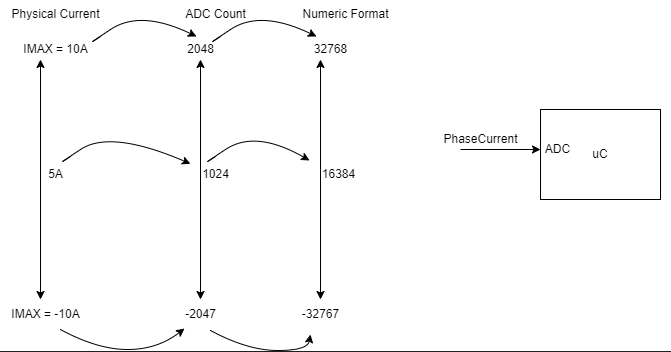# Per Unit Values understanding in FOC

I am really confused with per unit values used for currents, voltages in FOC programs, this is my understanding please correct me if i am wrong,For example i want to calculate the phase current reading
12 Bit ADC, +ve and -ve Currents

Step1: Calculate the maximum current possible for the system used. (10A in this example)
Step2: The maximum is assume 32768 (signed int value).
Step3: Calculate (1024/2048)*32768 = 16384.
Step4: Use 16384 value for other calculations example clarke transforms etc.

Oh wow, you’re asking complex questions. I think you will have to do quite a bit of reading/research to understand this well.

You should look into the concepts like:

• a calculation can use different units, like volts, milli-volts or kilo-volts
• a number can have different representations on the computer, like as an integer, or as a floating point number
• generally, for an algorithm working on numbers the answer will be correct if you use the right values in the right units
• but on the computer, especially when using the hardware like ADCs, you also have to consider the representation of numbers - some algorithms work on integers, while others use floating point numbers

So in answer to your example, I would say it is not completely correct.

• The ADC works with binary integer registers, so it returns a value like 1217 or 2067
• The meaning of these integers depends on several factors, like the configuration of the ADC, but also the external components used for the current sensing hardware
• So for sensing current you can generally say that you have an ADC resolution (in bits, representing the ADC sensitivity and therefore range of values you can read), ADC offset (representing the 0-value, because the current can flow in both directions) and an ADC gain (to convert the raw ADC value to a voltage), and a Volts to Amps ratio, (to then convert the volts to an amperage, since we are sensing current)
• So given an 10 bit ADC, it has range from 0-1023… if the ADC offset is 511, and the ADC gain is 100/V, and the Volts to Amps ratio is 0.5V/A, then reading a value of 621 from the ADC would mean:
(621 - 511) = 110 (amount above the 0V offset)
110/100 = 1.1V (this is voltage, based on the gain of 100/V)
1.1V / 0.5 = 2.2A (this is the amps, based on 0.5V/A)

Different algorithms may want different units, use different inputs, and some even work with only integer math. But SimpleFOC uses floating point math, and generally uses SI units like Volts, Amps, Ohms, Seconds and Radians for angles.